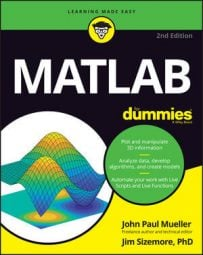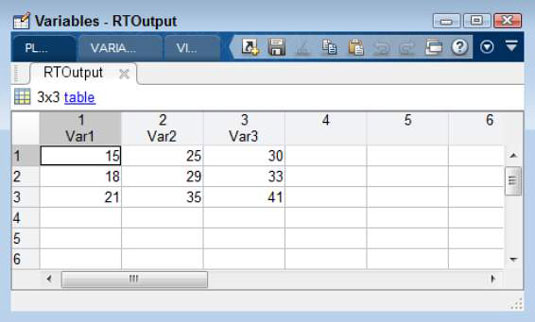##### MATLAB For DummiesA basic import uses all the default settings, which works fine for many kinds of data. MATLAB can determine the correct data format relatively often. An essential part of importing data is to use the correct import function. Each import function has features that make it more suitable to a particular kind of data. Here are some of the text-specific import functions and how they differ:

• csvread(): Works with numbers only, and the numbers must be separated by commas (hence the name Comma Separated Values, or CSV).

• dlmread(): Works with numbers only, but the numbers are normally separated by something other than commas.

• textscan(): Can import both numbers and strings. You must provide a format specification to read the data correctly.

• readtable(): Can import both numbers and strings. The output from this function is always a table, even when the source doesn’t contain tabular data.

The output you receive depends on the function you use. These examples each use a different method of reading the data from the disk. However, they all use the same data so that you can compare the results.

```15,25,30
18,29,33
21,35,41```

Using csvread() is the simplest option when working with data of this kind. All you do is type CSVOutput = csvread(‘NumericData.csv’) and press Enter. The output is a matrix that contains the following results:

```CSVOutput =
15 25 30
18 29 33
21 35 41```

The dlmread() function is a little more flexible than csvread() because you can supply a delimiter — a character used to separate values — as input. Each column is separated from the other by a comma. The rows are separated by a newline character. So, all you need to type in this case is DLMOutput = dlmread(‘NumericData.csv’); then press Enter. The output is a matrix containing these results:

```DLMOutput =
15 25 30
18 29 33
21 35 41```

## Using textscan()

The textscan() function can read both strings and numbers in the same data set. However, you must define a format specification to use this function. In addition, you can’t simply open the file and work with it. With these requirements in mind, you can use the following steps to help you use the textscan() function.

1. Type FileID = fopen(‘NumericData.csv’) and press Enter.

The textscan() function can’t open the file for you. However, it does accept the identifier that is returned by the fopen() function. The variable, FileID, contains the identifier used to access the file.

2. Type TSOutput = textscan(FileID, ‘%d,%d,%d/n’) and press Enter.

You get a single row of the data as output — not all three rows. In this case, the data is read into a cell array, not a matrix.

3. Type feof(FileID) and press Enter.

The function outputs a 0, which means that you aren’t at the end of the file yet. A simple test using the feof() function tells the loop to stop reading the file.

4. Type TSOutput = [TSOutput; textscan(FileID, ‘%f,%f,%f/n’)] and press Enter.

You now see the second row of data read in. These numbers are read as floating-point values rather than integers. Using textscan() gives you nearly absolute control over the appearance of the data in your application.

5. Type isinteger(TSOutput{1,1}) and press Enter.

The output value of 1 tells you that the element at row 1, column 1 is indeed an integer.

6. Type isinteger(TSOutput{2,1}) and press Enter.

This step verifies that the element at row 2, column 1 isn’t an integer because the output value is 0.

7. Type TSOutput = [TSOutput; textscan(FileID, ‘%2s,%2s,%2s/n’)] and press Enter.

8. Type textscan(FileID, ‘%d,%d,%d/n’) and press Enter.

This read should take you past the end of the file. The output is going to contain blank cells because nothing is left to read.

9. Type feof(FileID) and press Enter.

This time, the output value is 1, which means that you are indeed at the end of the file.

10. Type fclose(FileID) and press Enter.

MATLAB closes the file.

Failure to close a file can cause memory leaks and all sorts of other problems.

Now that you have a better idea of how a textscan() should work, it’s time to see an application that uses it.

```function [ ] = UseTextscan( )
%UseTextscan: A demonstration of the textscan() function
% This example shows how to use textscan() to scan
% the NumericData.csv file.
FileID = fopen(‘NumericData.csv’);
TSOutput = textscan(FileID, ‘%d,%d,%d/n’);
while not(feof(FileID))
TempData = textscan(FileID, ‘%d,%d,%d/n’);
if feof(FileID)
break;
end
TSOutput = [TSOutput; TempData];
end
disp(TSOutput);
fclose(FileID);
end```

Notice that you must verify that you haven’t actually reached the end of the file before adding the data in TempData to TSOutput. Otherwise, you end up with the blank row that textscan() obtains during the last read of the file.

The readtable() function works with both strings and numbers. It’s a lot easier to use than textscan(), but it also has a few quirks, such as assuming that the first row of data is actually column names. To use readtable() with the NumericData.csv file, type RTOutput = readtable(‘NumericData.csv’, ‘ReadVariableNames’, false) and press Enter. You see the following output:

```RTOutput =
Var1 Var2 Var3
____ ____ ____
15  25  30
18  29  33
21  35  41```

The output actually is a table rather than a matrix or a cell array. The columns have names attached to them. As a consequence, you can access individual members using the variable name, such as RTOutput{1, ‘Var1’}, which outputs a value of 15 in this case.Notice that readtable() accepts property name and value pairs as input. In this case, ‘ReadVariableNames’ is a property. Setting this property to false means that readtable() won’t read the first row as a heading of variable names. You use readtable() where the output file does contain variable names because having them makes accessing the data easier in many situations.